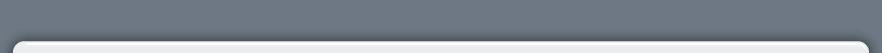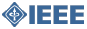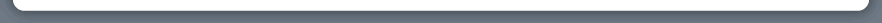IEEE Home | Shop IEEE | Join IEEE | myIEEE | Contact IEEE | IEEEXploreIEEE Student Branch - Faculty of Engineering University of PeradeniyaMain Menu
 » University of Peradeniya» Faculty of Engineering» Reservation & Articles Upload» Articles» Downloads» Gallery» MessagesHome>Articles

Articles

1) How to Apply Capacitors to Low Voltage Power Systems

UNDERSTANDING POWER FACTOR

In most modern electrical distribution systems, the predominant loads are resistive and inductive. Resistive loads are incandescent lighting and resistance heating. Inductive loads are A.C. Motors, induction furnaces, transformers and ballast-type lighting. Inductive loads require two kinds of power:  (1) active (or working) power to perform the work (motion) and (2) reactive power to create and maintain electro-magnetic fields. The vector sum of the active power and reactive power make up the total (or apparent) power used. This is the power generated by the utility for the user to perform a given amount of work.

* Active power is measured in KW (1000 Watts)
* Reactive power is measured in KVAR (1000 Volt-Amperes Reactive)
* Total Power is measured in KVA (1000 Volts-Amperes)

Power factor then is the ratio of active power to total power. We can illustrate these relationships by means of a right triangle. (See Figure 1.)Note that a low power factor requires a larger amount of KVA to accomplish a fixed amount of work (KW), whereas a high power factor would require a lesser amount of KVA to accomplish the same amount of work.  Utilities provide the KVA to the user, and by means of continuous metering, they bill the user each month, and provide actual values of the components of power shown in Figure 1.  If the values shown on the bill indicate a low power factor, many utilities will add a penalty to the bill. In like manner, a high power factor may result in a reduction in the over-all cost of total power consumed.

IMPROVING POWER FACTOR

The solution is to add power factor correction capacitors to the plant power distribution system.  They act as reactive power generators, and provide the needed reactive power to accomplish KW of work. This then reduces the amount of reactive power, and thus total power, generated by the utility.  Let’s look at an actual case of power factor improvement to an industrial plant, and the savings that resulted.  (See Figure 2)Because the utility applied a penalty formula when the power factor fell below 85%, this user had a penalty of Rs.650.00 added to the bill.  To accomplish 812KW of work the 1500KVA
Transformer was almost 78% loaded. (1160÷1500 = 77.3%). The solution in this case was to add capacitors to the system by installing them at each of thirteen large motors. The total KVAR added was 410. This improved the power factor to 89%, and reduced the required KVA to 913, which is the vector sum of KW and KVAR.  (See Figure 3)The user, doing the same amount of work, but now with capacitors installed, has eliminated the Rs.650.00 monthly penalty. This would be an annual savings of Rs.7800.00.  The capacitors and the labour to install them cost Rs.7351.00, a payback of less than 12 months.

The utility has to generate 247 less KVA (1160 - 913 = 247), and the user has the 1500 KVA transformer now loaded only to 60% of capacity.  This will allow the addition of more loads in the future to be supplied by the transformer.

ELIMINATION OF PENALTY MONEY

A high power factor eliminates penalty money imposed when operating with a low power factor.  For many years, most utilities demanded a minimum of 85% power factor as an average for each monthly billing.  Now many of these same utilities are demanding 95%...or else pay a penalty!

The actual wording or formula in the utility rate contract might spell out the required power factor, or it might refer to KVA billing, or it might refer to KW demand billing with power factor adjustment multipliers.  Have your utility representative explain the particular rate contract used in your monthly bill.  This will insure you are taking the proper steps to obtain maximum dollar savings by maintaining a proper power factor.

ADDITIONAL CAPACITY IN ELECTRICAL SYSTEM

A high power factor can help you utilize the full capacity of your electrical system. To refresh our memory, let’s look again at the power triangle story, shown on Pages 3 & 4, Figures 1, 2, and 3.  Remember that KVA is a measure of the total power generated by the utility for you to accomplish your KW of work. Remember that the KVA figure is the amount of power passing through your plant transformer, and limited by its rated size:  e.g. 750 KVA, 1500 KVA, 2500 KVA, etc.  In the previous example, we reduced your transformer loading from 1160 to 913 KVA, thus allowing for more load to be added in the future.

To download this article click here >>>

W. M. I. P. Divarathne,
E /05 Batch,
e05isurupdn@gmail.com,
+94-77-2332787.Home   |    IEEE Home   |    University of Peradeniya   |    Faculty of Engineering   |    Privacy & Security   |    Terms & Conditions